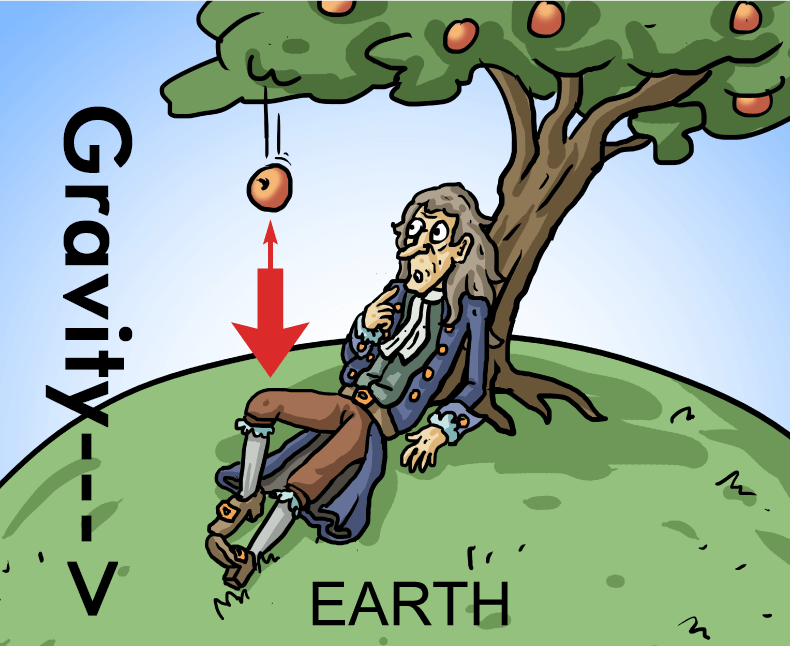# Gravitational Force Calculator

Enter values in given input box to find force of gravity by using our Gravitational Force Calculator.

Formula F = G m1 m2## Gravitational Force Equation / Formula

Gravitational Force :
F = Gm1m2 r2

Mass of Object 1 :
m1 = Fr2 Gm2

Mass of Object 2 :
m2 = Fr2 Gm1

Distance between the Objects :

r = Gm1m2F

Where, G = Universal Gravitational Constant = 6.6726 x 10-11N-m2/kg2
m1 =Mass of Object 1
m2 =Mass of Object 2
r = Distance Between the Objects.

The force of gravity calculator finds the force of gravity using Newton’s Law of gravity. Gravity calculator is mainly used in physics. It saves your time by calculating the following terms in one place:

• Gravitational force F
• Mass of 1st object m1
• Mass of 2nd object m2
• Distance between objects r

Let’s explore gravitational force definition, formula of gravitational force, and how to find gravitational force without using gravity force calculator.

## What is gravitational force?

Newton’s Law of Gravity states that two particles attract each other with forces. This principle is directly proportional to the product of their masses which is divided by the square of the distance between the particles.

In short, when two bodies attract each other with equal and opposite forces, it is said to be the gravitational force. It is basically a force of attraction. It is denoted by the symbol “F”. The SI unit of gravitational force is Newton Meter (N).This Newton’s law of gravity calculator is used to calculate the gravitational force between two objects when mass and distance between the objects are known.

## How to calculate gravitational force?

Although, the gravitational constant calculator eases up the gravity calculation, however, you should know how to find the force of gravity by yourself. Gravitational attraction calculator brings much-needed convenience, but you should know the method especially if you are a student.

Example:
Calculate the force of gravity if mass of first object is 5 kg, mass of second object is 10 kg, and the distance between both objects is 15 m.

Solution:

Step 1: Identify and write down the values.

m1 = 5 kg
m2 = 10 kg
r = 15 m

Step 2: Use the gravity equation and place the values.
F = Gm1m2 r2

Since, G = 6.6726 x 10-11 N-m2/kg2
F = (6.6726 x 10-11×5×10)/152
F = 1.4828 × 10-11 N

### References:

1. What Is Gravity? | NASA Space Place from, spaceplace.nasa.gov.
2. Gravitational Force - an overview | ScienceDirect Topics.
3. What is Gravitational Force? - Universe Today.
4. Define gravity with example? Retrieved from starchild.gsfc.nasa.gov.